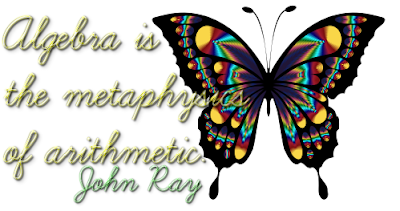## Linear Equations In One Variable

Class 8 Maths

#### Q & A based on Class 8 NCERT Chapter

Q1(MCQ): If 3x – 4 (64 – x) = 10, then the value of x is

(a) –266
(b) 133
(c) 66.5
(d) 38

3x - 256 + 4x =10
7x = 10 + 256 = 266
x = 266/7 = 38

Q2: Three consecutive even numbers whose sum is 156 are 51, 52 and 53.
State if the above statement is True or False

Let the three consecutive even numbers be x, x+2, x+4
∴ x + x+ 2 + x+4 = 156
⇒ 3x + 6 = 156
⇒ 3x = 156 - 6 = 150
⇒ x = 150 /  3 = 50
Thus numbers are 50, 51, 52

Q3: Fifteen added to thrice a whole number gives 93. The number is __________.

Q4: x = –12 is the solution of the linear equation 5x –3(2x + 1) = 21+x (True or False)

For x = -12,
LHS = 5(-12) -3[2(-12) + 1] = -60 -3[-24+ 1]  = -60 -3(23) = -60 + 69 = 9
RHS = 21 + (-12) = 21 - 12 = 9
Thus LHS =  RHS

Q5: The present age of a father is four times the age of his son. After 10 years, the age of father will become three times the age of his son. Find their present ages.

Answer: Let the present age of son be x years
∴ The present age of father = 4x years
After 10 years,
Age of son = (x + 10) years
Age of father = (4x + 10) years

According to the given condition
4x + 10 = 3(x + 10)
4x + 10 = 3x + 30
4x – 3x = 30 –10
x = 20
∴ Present age of son = 20 years.
and present age of father = 4x = 4 × 20 = 80 years.

Q6(MCQ): If 8x – 3 = 25 +17x, then x is

(a) a fraction
(b) an integer
(c) a rational number
(d) cannot be solved

8x - 3 = 25 + 17x
On transposing,
8x - 17x = 25 + 3
-9x = 28
x = -28/9  which is a rational number.

Q7(MCQ): The shifting of a number from one side of an equation to other is called

(a) Transposition
(b) Distributivity
(c) Commutativity
(d) Associativity

Q8(MCQ): A linear equation in one variable has

(a) Only one solution
(b) Two solutions
(c) More than two solutions
(d) No solution

Q9(MCQ): The sum of three consecutive multiples of 7 is 357. Find the smallest multiple.

(a) 112
(b) 126
(c) 119
(d) 116

Let the numbers be x, x+7, x+14
∴ x + x + 7 + x + 14 =  357
⇒ 3x + 21 = 357
⇒ 3x = 357 - 21 = 336
⇒ x  336 / 3 = 112

Q10: The solution of the equation 3x – 4 = 1 – 2 x is _________.

Q11: In a linear equation, the _________ power of the variable appearing in the equation is one.

Q12: Rs. 13500 are to be distributed among Salma, Kiran and Jenifer in such a way that Salma gets Rs. 1000 more than Kiran and Jenifer gets Rs. 500 more than Kiran. Find the money received by Jenifer.

Let Kiran gets = ₹p
Salma gets - ₹p + 1000
Jenifer gets = ₹p + 500
∴ Sum is p + p + 1000 + p + 500 = 13500
⇒ 3p = 13500 - 1500 = 12000
⇒ p = 12000/3 = 4000
∴ Jenifer gets = 4000 + 500 = ₹ 45001.This comment has been removed by the author.

2.Comment....

3.answer of question 4 is wrong

1.Thanks Ojas for finding the error. It has been corrected. Thanks.

2.This actually not a a type of m. C.q. in m. C. Q there r basic concepts so you should post another m. C. Q

4.the answer is still not corrected

1.Typo corrected in Q4.

5.no it is right

6.then what is the correct answer